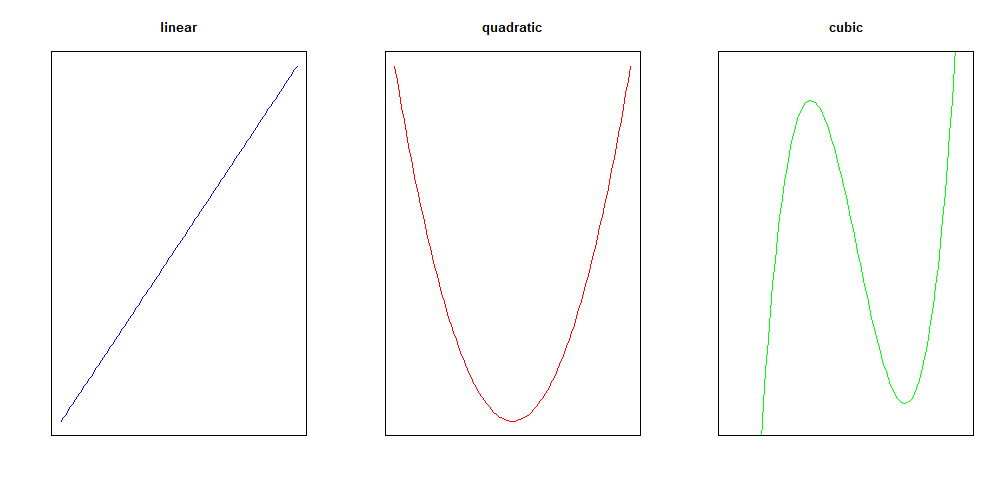# Some models have curves

Directions: Follow along with the slides, completing the questions in blue on your computer, and answering the questions in red in your journal.

Space, Click, Right Arrow or swipe left to move to the next slide.

# Making models do yoga

• So far, we have only worked with prediction models that fit the line of best fit to the data.
• What happens if the true relationship between the data is nonlinear?
• In this lab, we will learn about prediction models that fit best fitting curves to data.
• Before moving on, load the movie data and split it into two sets:
• A set named training that includes 75% of the data.
• And a set named testing that includes the remaining 25%.
• Remember to use set.seed.

# Problems with lines

• Before learning how to fit curves, let’s first fit a linear model for reference.
• Train a linear model predicting audience_rating based on critics_rating for the training data. Assign this model to movie_linear.
• Fill in the blanks below to create a scatterplot with audience_rating on the y-axis and critics_rating on the x-axis using your testing data.
xyplot(____ ~ ____, data = ____)
• Previously, you used add_line to plot the line of best fit. An alternative function for plotting the line of best fit is add_curve, which takes the name of the model as an argument.
• Run the code below to add the line of best fit for the training data to the plot.
add_curve(movie_linear)
• Describe, in words, how the line fits the data. Are there any values for critics_rating that would make obviously poor predictions?
• Hint: how does the linear model perform on very low and very high values of critics_rating?
• Compute the MSE of the linear model for the testing data and write it down for later.
• Hint: refer to lab 4B.

• You don’t need to be a full-fledged Data Scientist to realize that trying to fit a line to curved data is a poor modeling choice.
• If our data is curved, we should try to model it with a curve.
• Instead of fitting a line, with equation of the form
y = a + bx
• we might consider fitting a quadratic curve, with equation of the form
y = a + bx + cx2
• or even a cubic curve, with equation of the form
y = a + bx + cx2+ dx3
• In general, the more coefficients in the model, the more flexible its predictions can be.# Making bend-y models

• To fit a quadratic model in R, we can use the poly() function.
• Fill in the blanks below to train a quadratic model predicting audience_rating from critics_rating, and assign that model to movie_quad.
movie_quad <- lm(____ ~ poly(____, 2), data = training)
• What is the role of the number 2 in the poly() function?

# Comparing lines and curves

• Fill in the blanks below to
• create a scatterplot with audience_rating on the y-axis and critics_rating on the x-axis using your testing data, and
• add the line of best fit and best fitting quadratic curve.
• Hint: the col argument is added to the add_curve functions to help distinguish the two curves.
xyplot(____ ~ ____, data = ____)
add_curve(____, col = "red")
• Compare how the line of best fit and the quadratic model fit the data. Which do you think has a lower test MSE?
• Compute the MSE of the quadratic model for the test data and write it down for later.
• Use the difference in each model’s test MSE to describe why one model fits better than the other.
• Create a model that predicts audience_rating using a cubic curve (polynomial with degree 3), and assign this model to movie_cubic.
• Create a scatterplot with audience_rating on the y-axis and critics_rating on the x-axis using your test data.
• Using the names of the three models you have trained, add the line of best fit, best fitting quadratic curve, and best fitting cubic curve for the training data to the plot.
• Based on the plot, which model do you think is the best at predicting the testing data?
• Use the difference in testing MSE to verify which model is the best at predicting the testing data.